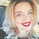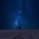2790 obejrzeń
RSI of MAs is designed to calculate the moving average for a specific period, and then take the RSI of that value. This script allows the user to select which moving average they would like to utilize for the calculation, as well as customizing how the Signal Line is calculated. There are many combinations available and you will need to tune the indicator to fit your trading style. The Signal Line is designed to indicate when there is a potential change in price action. If the Signal Line is below RSIoMA, price is bullish . If the Signal Line is above RSIoMA, price is bearish .

MA Period is the length/period the moving average is calculated with
RSI Period is the length/period the RSI is calculated with
RSI MA Mode determines which moving average is applied to the MA period

Signal Line determines which moving average or QQE is used to calculate the signal line
Signal Line Period is the length/period the Signal Line is calculated with

Multiple MA Options Credits to @Fractured
Signal Line Options Credits to @lejmer
Bits and Pieces from @AlexGrover, @Montyjus, and @Jiehonglim

## Komentarzeraiinzen
@raiinzen, I did not code in an alerts system. You can interpret the information however you like but it's set up to be utilized when you are actively trading.
OdpowiedzHello eylwithsteph

i want to write one small script for RSI did you provide this services
Odpowiedz